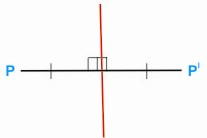HOME MATH DICTIONARY DOWNLOAD FEEDBACK DISCLAIMER
 Question: What is Reflection ? Answer: A reflection is a transformation which has the same effect as a mirror. Every point P in front of a mirror (line or plane) maps to an image point P' on the other side of the mirror so that P’ and P are the same distance from the mirror, and PP’ is at right angles to it.For example, a reflection of the small English letter p in respect to a vertical line would look like q. In order to reflect a planar figure one needs the mirror to be a line (axis of reflection), while for reflections in the three-dimensional space one would use a plane for a mirror. Reflection sometimes is considered as a special case of inversion with infinite radius of the reference circle. Geometrically, to find the reflection of a point one drops a perpendicular from the point onto the line (plane) used for reflection, and continues the same distance on the other side. To find the reflection of a figure, one reflects each point in the figure. The effect on any object is to produce an image of the same shape and size but in the opposite sense. The matrix for reflection in a line through the origin at an angle α to the x-axis is Cos 2α Sin 2α Sin 2α - Cos 2α